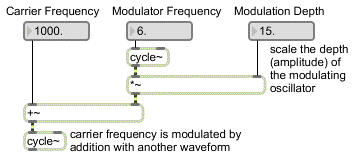Tutorial 10: Vibrato and FM
Basic FM in MSP
Frequency modulation (FM) is a change in the frequency of one signal caused by modulating it with another signal. In the most common implementation, the frequency of a sinusoidal carrier wave is varied continuously with the output of a sinusoidal modulating oscillator. The modulator is added to the constant base frequency of the carrier.Simple frequency modulation
The example above shows the basic configuration for FM. The frequency of the modulating oscillator determines the rate of modulation, and the amplitude of the modulator determines the ‘depth’ (intensity) of the effect.
• Click on the ezdac~ to turn audio on.
The sinusoidal movement of the modulator causes the frequency of the carrier to go as high as 1015 Hz and as low as 885 Hz. This frequency variation completes six cycles per second, so we hear a 6 Hz vibrato centered around 1000 Hz. (Note that this is distinct from tremolo, which is a fluctuation in amplitude, not frequency.)
• Drag upward on the number box marked ‘Modulation Depth’ to change the amplitude of the modulator. The vibrato becomes wider and wider as the modulator amplitude increases. Set the modulation depth to 500.
With such a drastic frequency modulation, one no longer really hears the carrier frequency. The tone passes through 1000 Hz so fast that we don't hear that as its frequency. Instead we hear the extremes -- 500 Hz and 1500 Hz -- because the output frequency actually spends more time in those areas.
Note that 500 Hz is an octave below 1000 Hz, while 1500 Hz is only a perfect fifth above 1000 Hz. The interval between 500 Hz and 1500 Hz is thus a perfect 12th (as one would expect, given their 1:3 ratio). So you can see that a vibrato of equal frequency variation around a central frequency does not produce equal pitch variation above and below the central pitch. (In Tutorial 17 we demonstrate how to make a vibrato that is equal in pitch up and down.)
• Set the modulation depth to 1000. Now begin dragging the ‘Modulator Frequency’ number box upward slowly to hear a variety of effects.
As the modulator frequency approaches the audio range, you no longer hear individual oscillations of the modulator. The modulation rate itself is heard as a low tone. As the modulation frequency gets well into the audio range (at about 50 Hz), you begin to hear a complex combination of sidebands produced by the FM process. The precise frequencies of these sidebands depend on the relationship between the carrier and modulator frequencies.
• Drag the ‘Modulator Frequency’ number box all the way up to 1000. Notice that the result is a rich harmonic tone with fundamental frequency of 1000 Hz. Try typing in modulator frequencies of 500, 250, and 125 and note the change in perceived fundamental.
In each of these cases, the perceived fundamental is the same as the modulator frequency. In fact, though, it is not determined just by the modulator frequency, but rather by the relationship between carrier frequency and modulator frequency. This will be examined more in the next chapter.
• Type in 125 as the modulator frequency. Now drag up and down on the ‘Modulation Depth’ number box, making drastic changes. Notice that the pitch stays the same but the timbre changes.
The timbre of an FM tone depends on the ratio of modulator amplitude to modulator frequency. This, too, will be discussed more in the next chapter.
Summary
Frequency modulation (FM) is achieved by adding a time-varying signal to the constant frequency of an oscillator. It is good for vibrato effects at sub-audio modulating frequencies, and can produce a wide variety of timbres at audio modulating frequencies. The rich complex tones created with FM contain many partials, even though only two oscillators are needed to make the sound. This is a great improvement over additive synthesis, in terms of computational efficiency.